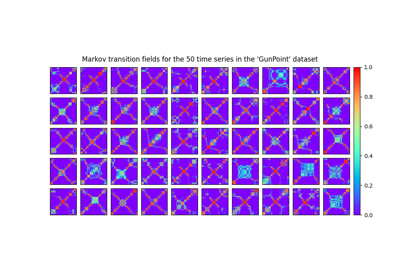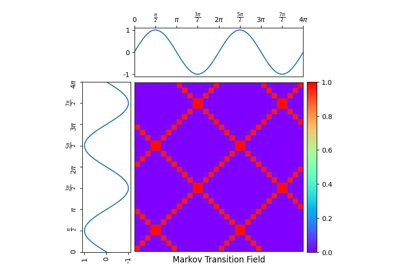# `pyts.image`.MarkovTransitionField¶

class `pyts.image.``MarkovTransitionField`(image_size=1.0, n_bins=8, strategy='quantile', overlapping=False, flatten=False)[source]

Markov Transition Field.

Parameters: image_size : int or float (default = 1.) Shape of the output images. If float, it represents a percentage of the size of each time series and must be between 0 and 1. Output images are square, thus providing the size of one dimension is enough. n_bins : int (default = 5) Number of bins (also known as the size of the alphabet) strategy : ‘uniform’, ‘quantile’ or ‘normal’ (default = ‘quantile’) Strategy used to define the widths of the bins: ‘uniform’: All bins in each sample have identical widths ‘quantile’: All bins in each sample have the same number of points ‘normal’: Bin edges are quantiles from a standard normal distribution overlapping : bool (default = False) If False, reducing the image with the blurring kernel will be applied on non-overlapping rectangles. If True, it will be applied on possibly overlapping squares. flatten : bool (default = False) If True, images are flattened to be one-dimensional.

References

  Z. Wang and T. Oates, “Encoding Time Series as Images for Visual Inspection and Classification Using Tiled Convolutional Neural Networks.” AAAI Workshop (2015).

Examples

```>>> from pyts.datasets import load_gunpoint
>>> from pyts.image import MarkovTransitionField
>>> X, _, _, _ = load_gunpoint(return_X_y=True)
>>> transformer = MarkovTransitionField()
>>> X_new = transformer.transform(X)
>>> X_new.shape
(50, 150, 150)
```

Methods

 `__init__`([image_size, n_bins, strategy, …]) Initialize self. `fit`([X, y]) Pass. `fit_transform`(X[, y]) Fit to data, then transform it. `get_params`([deep]) Get parameters for this estimator. `set_params`(**params) Set the parameters of this estimator. `transform`(X) Transform each time series into a MTF image.
`__init__`(image_size=1.0, n_bins=8, strategy='quantile', overlapping=False, flatten=False)[source]

Initialize self. See help(type(self)) for accurate signature.

`fit`(X=None, y=None)[source]

Pass.

Parameters: X Ignored y Ignored self : object
`fit_transform`(X, y=None, **fit_params)

Fit to data, then transform it.

Fits transformer to X and y with optional parameters fit_params and returns a transformed version of X.

Parameters: X : array-like, shape = (n_samples, n_timestamps) Univariate time series. y : None or array-like, shape = (n_samples,) (default = None) Target values (None for unsupervised transformations). **fit_params : dict Additional fit parameters. X_new : array Transformed array.
`get_params`(deep=True)

Get parameters for this estimator.

Parameters: deep : bool, default=True If True, will return the parameters for this estimator and contained subobjects that are estimators. params : dict Parameter names mapped to their values.
`set_params`(**params)

Set the parameters of this estimator.

The method works on simple estimators as well as on nested objects (such as `Pipeline`). The latter have parameters of the form `<component>__<parameter>` so that it’s possible to update each component of a nested object.

Parameters: **params : dict Estimator parameters. self : estimator instance Estimator instance.
`transform`(X)[source]

Transform each time series into a MTF image.

Parameters: X : array-like, shape = (n_samples, n_timestamps) Input data X_new : array-like, shape = (n_samples, image_size, image_size) Transformed data. If `flatten=True`, the shape is (n_samples, image_size * image_size).

## Examples using `pyts.image.MarkovTransitionField`¶Data set of Markov transition fieldsSingle Markov transition field